Microsoft Excel quick and easy

New Excel tutorial every week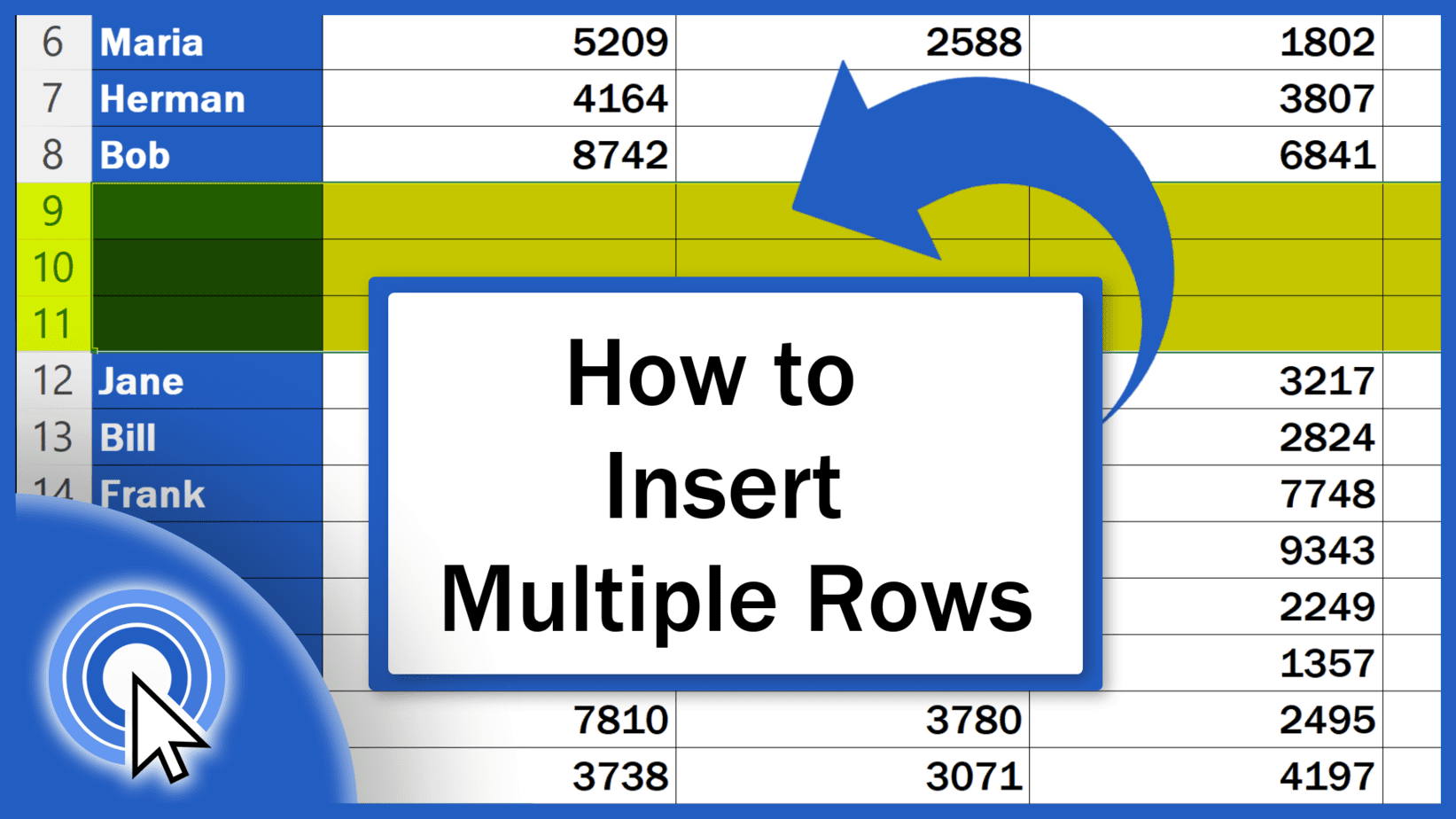## How to Insert Multiple Rows in Excel (The Simplest Way)

In this tutorial, we’ll be looking at the simplest way how to insert multiple rows in Excel at once. Let’s have a look now!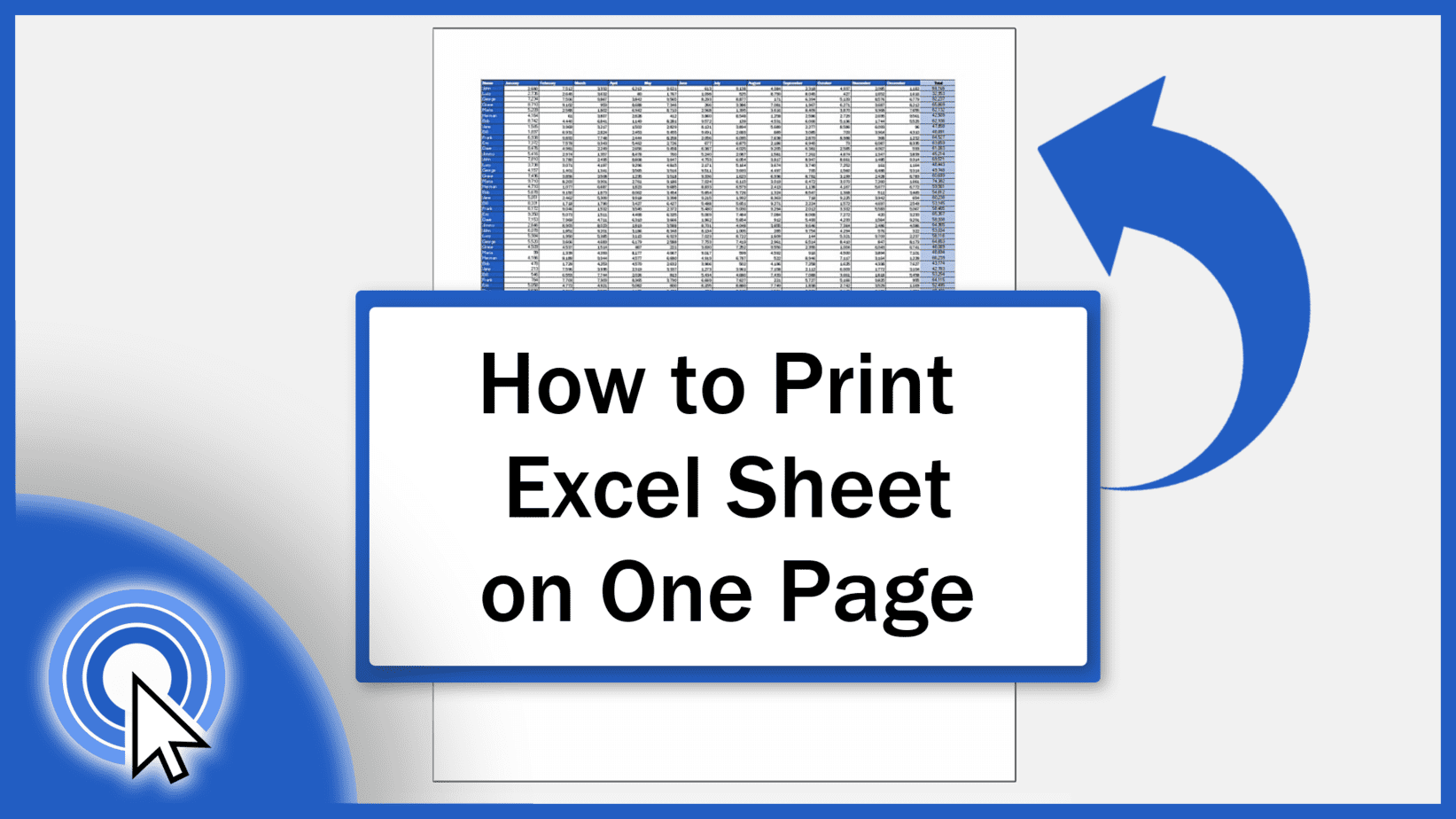## How to Print an Excel Sheet on One Page (the Simplest Way)

Today we’ll go through the simplest way how to print an Excel sheet on one page. Let’s start!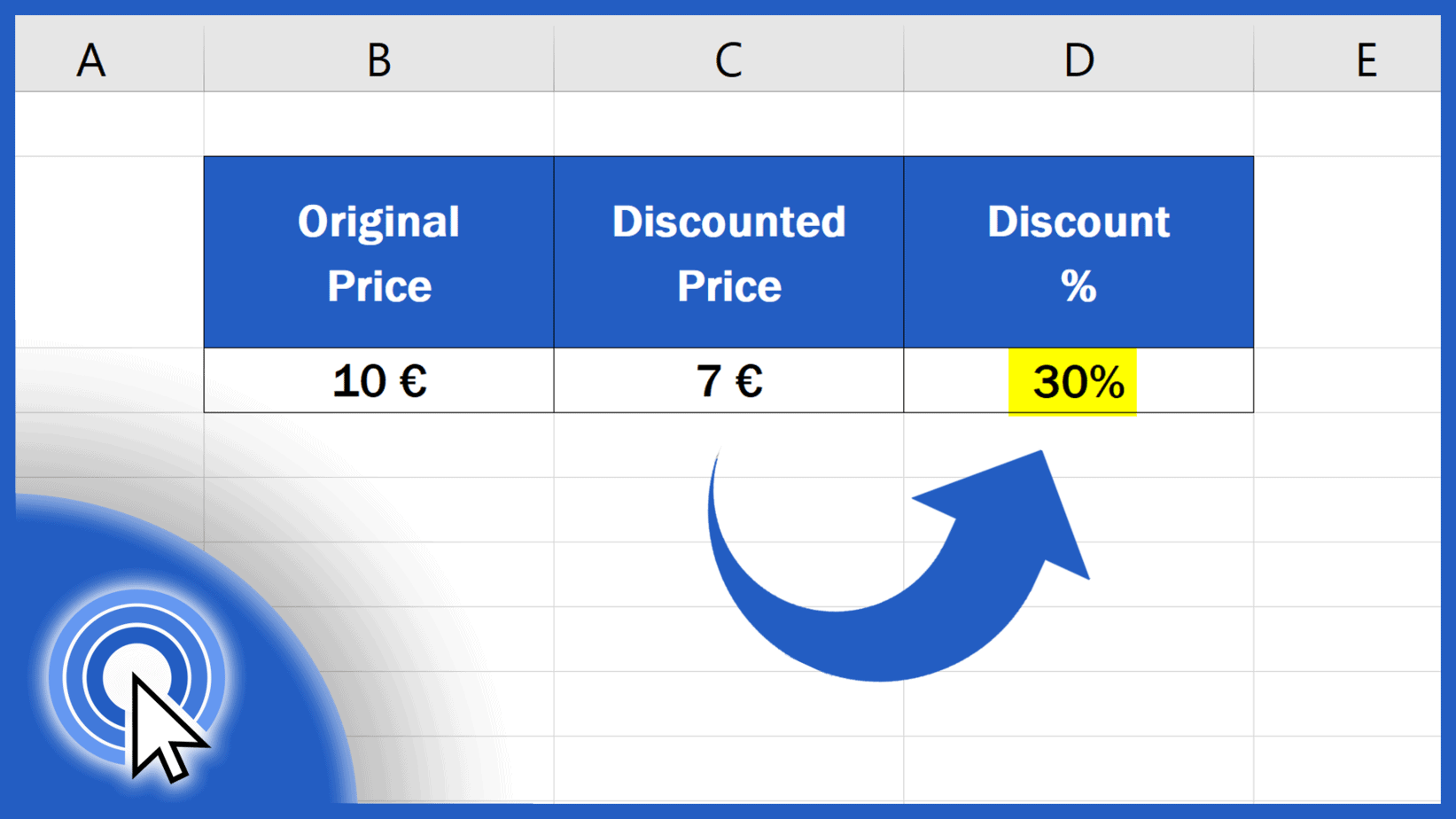## How to Calculate Discount Percentages in Excel (Quick and Easy)

Today we’ll be talking about how to calculate discount percentages in Excel in a quick and easy way. Let’s get into it right now!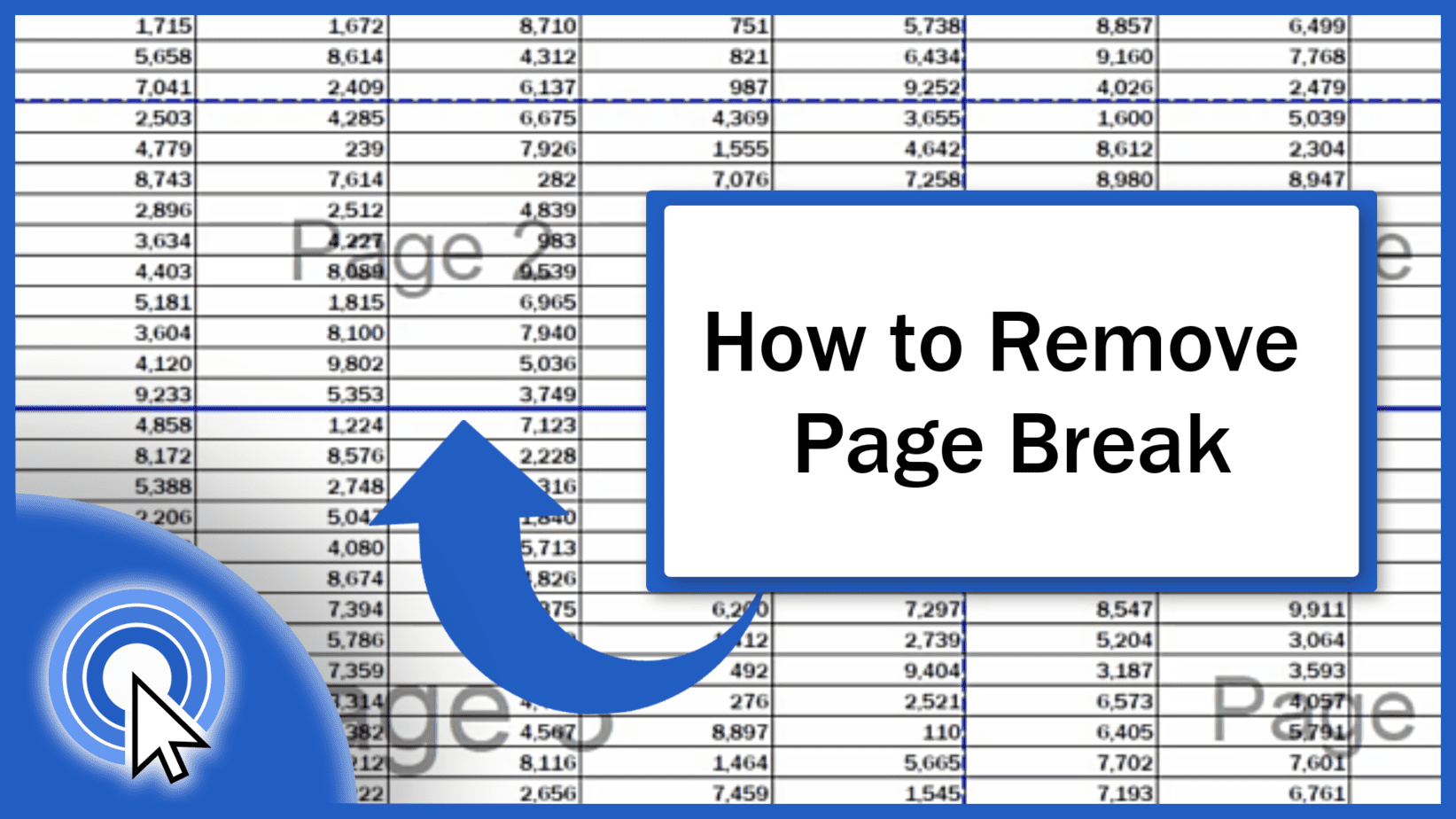## How to Remove a Page Break in Excel (One by One or All at Once)

In today’s tutorial, we’re going to have a look at how to remove a page break in Excel – the horizontal, the vertical or all of them at once.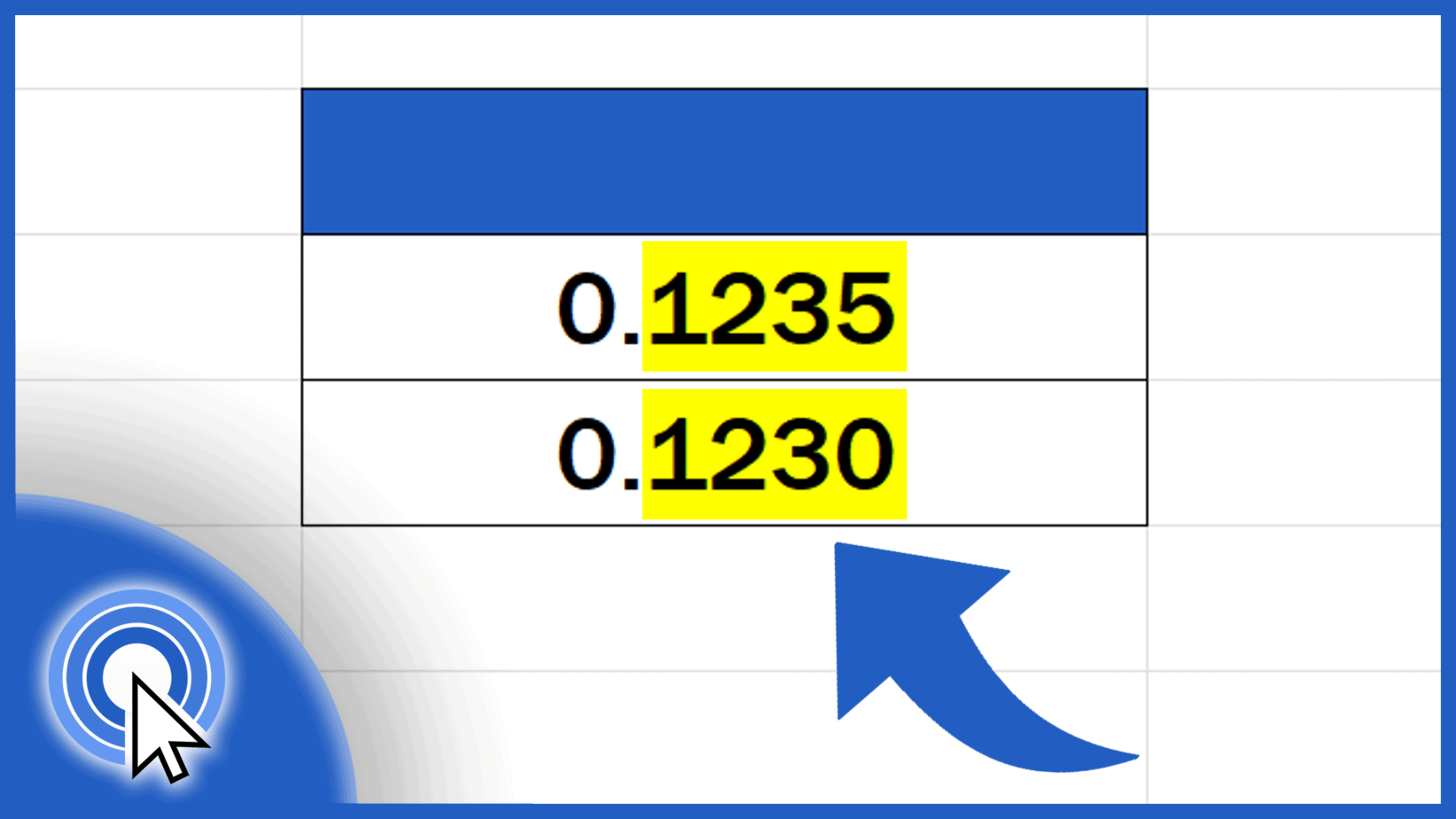## How to Change the Number of Decimal Places in Excel (Two Most Common Ways)

Today we’re going to talk about two most common ways how to change the number of decimal places in Excel. Ready to start?## How to Write the Squared Symbol in Excel (within Text and as Numbers)

This tutorial offers a step-by-step guide on how to write the squared symbol in Excel. We’ll have a look at two ways to do this.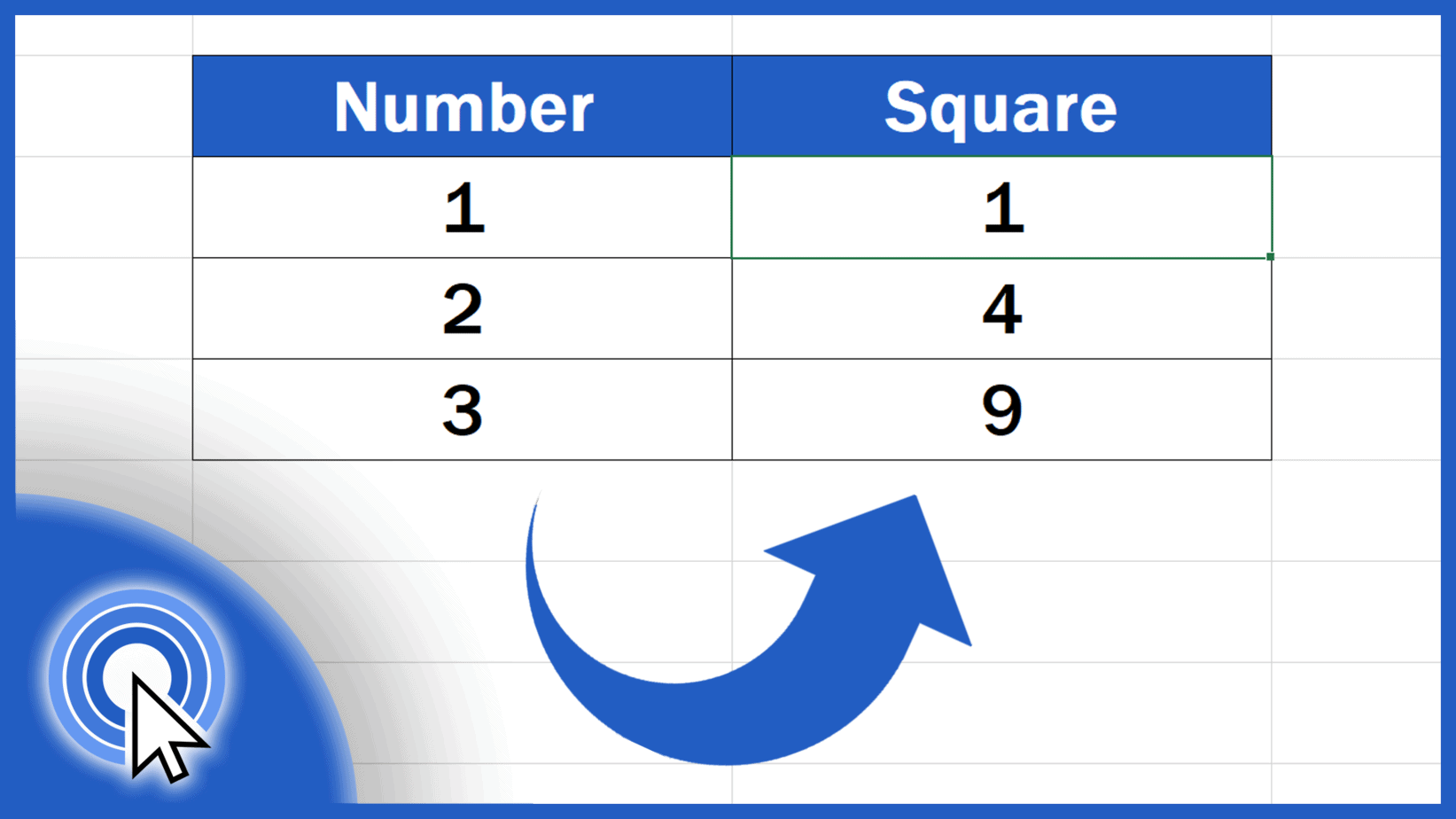## How to Square a Number in Excel (Two Most Common Ways)

In this tutorial we’re going to go through two most common ways how to square a number in Excel. Keep watching to learn more!## How to Set the Print Area in Excel (Step by Step)

Today we’ll be talking about how to set the print area in Excel. Keep watching and print any part of your Excel sheet without a hitch!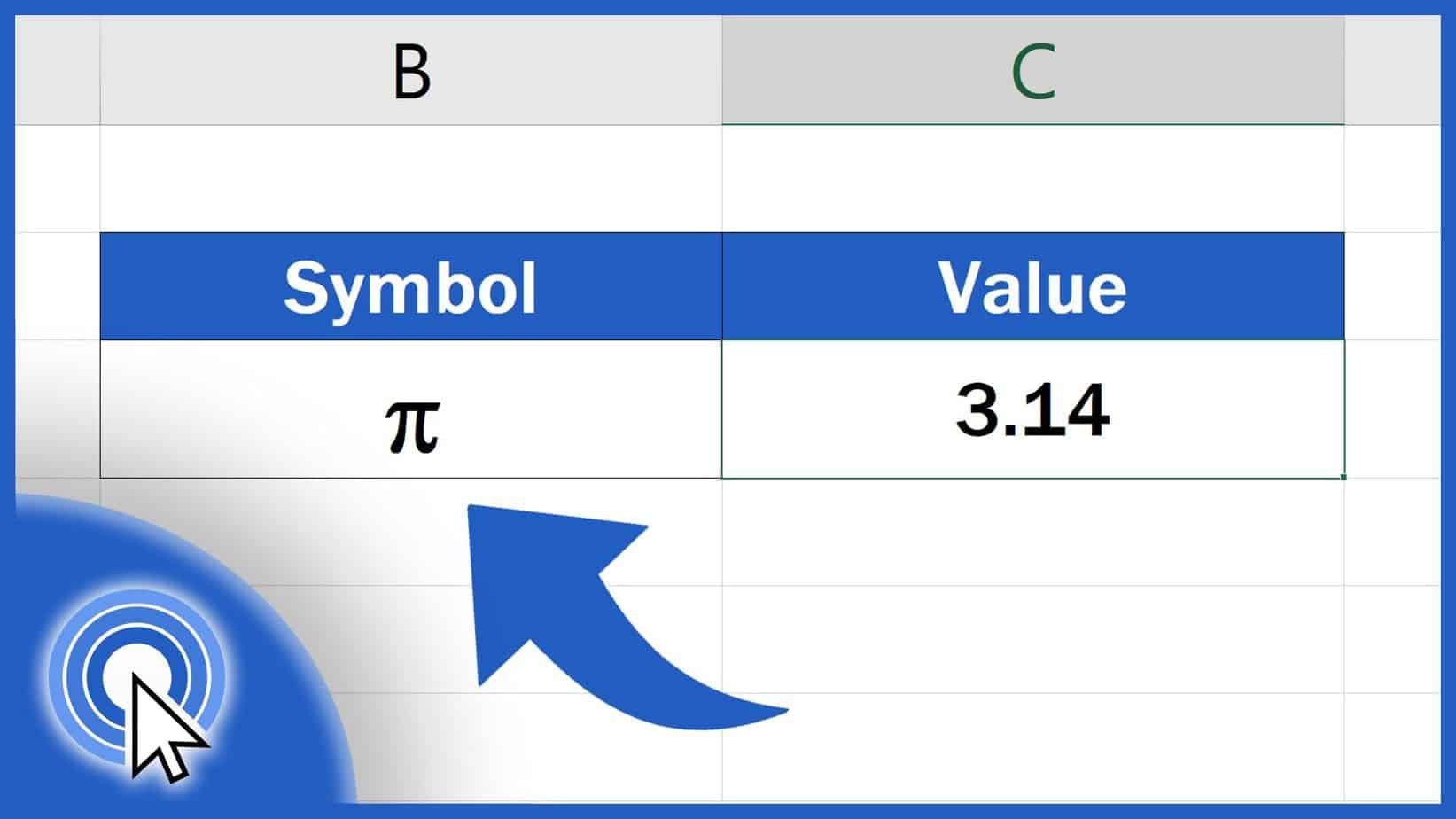## How to Write Pi in Excel (including the Value)

Today we’ll have a look at how to write the Pi symbol and get its exact value in a cell.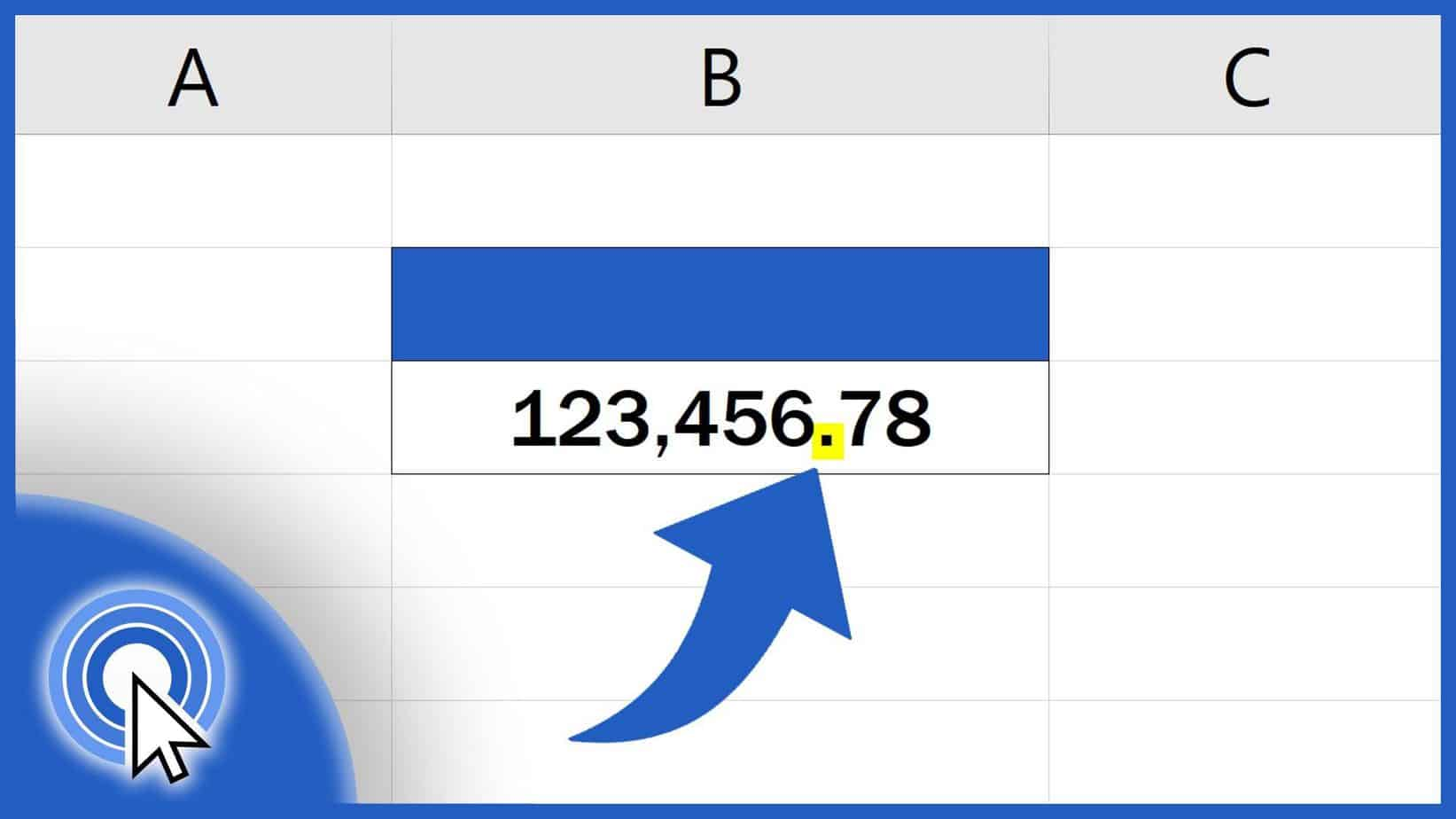## How to Change the Decimal Separator in Excel (including the Thousands Separator)

Here we are with a super quick way how to change the decimal (and thousands) separator in Excel if needed.